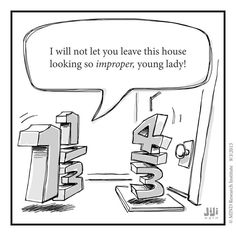## Math at a Glance Week of October 29thHappy Halloween!

This week in Middle School Math, we are:

6th Grade – Section A – Mrs. VonFeldt  This week we continue to use negative integers and rational numbers. Students will learn about absolute value and how to use it in calculations. We will learn about graphing on the coordinate system and how to find the distance between two points. Please see the Khan Academy link in Mrs. Evan’s post about investigating the coordinate system.

6th Grade – Section B – Mrs. Evans

This week we are continuing with Chapter 3 in which we are becoming familiar with negative integers and rational numbers.  We will learn about absolute value and the coordinate system.  Here is a great Khan Academy link to introduction to the coordinate system for you to investigate:

https://www.khanacademy.org/math/basic-geo/basic-geo-coord-plane/coordinate-plane-quad-1/v/introduction-to-the-coordinate-plane

Pre Algebra (7th and 8th Grade) – Mrs. Evans

This week we will finish up Unit 1 testing and begin Module 4 in which we will investigate rates and unit rates.  We will be able to see how useful unit rates are when we are comparing two rates.  We will also begin to look at proportional or constant rates and how we can manipulate them.   You can help your student to understand this topic by observing in every day life where you use a rate like \$/lb or miles/hour.  Examples of this surround us!  If you shop together, challenge your student to compare two like things by using unit rates.

Algebra – Mrs. VonFeldt

This week the class is continuing to graph linear equations. We will discuss when it is easier to graph a line using the intercepts and when it is easier to use the formula y = mx + b. Students will be able to rewrite equations to translate from one form to the other. Function notation will be introduced. There will be a Chapter 3 Test this Friday. Below is a fun video to help remember the y = mx + b formula.

## Math at a Glance Week of Oct 22ndHappy Autumn!  This week in Middle School Math, we are:

6th Grade – Section A – Mrs. VonFeldt  This week we begin Chapter 3. We will explore negative numbers and what they represent in real life. The students will start their number line project which involves working collaboratively with a team to create a large number line and plot a set of rational numbers.

6th Grade – Section B – Mrs. Evans

This week we will be moving on to Chapter 3 in which we will explore negative numbers, how to plot and compare them and what they represent in real life.  We will be applying what we learn to integers and rational numbers.

Pre Algebra (7th and 8th Grade) – Mrs. Evans

This week we will finish up Unit 1 with the application of all operations of rational numbers.  We will practicing multi step solving word problems by developing a plan.   We will have our Unit 1 test on Friday.

For those who would like to have additional practice with the addition and subtraction of negative and positive numbers can visit the Khan Academy link below.  There are videos about it as well as on line practice.

https://www.khanacademy.org/math/cc-seventh-grade-math/cc-7th-negative-numbers-add-and-subtract

Algebra – Mrs. VonFeldtThis week the students are exploring slope as a rate of change. We will calculate slope when given either a graph or two points. We will also use the slope and y-intercept to quickly write an equation and graph a line.

## Math Rocks!

Welcome to our first blog entry!

We are so pleased to have this great avenue to communication about what our classes are doing in math!  We hope that you find it a place for information, ideas and communication.

This week in Middle School Math, we are:

6th Grade – Section A – Mrs. VonFeldt  This week we will continue to multiply and divide fractions and mixed numbers. Students will create a journal entry that reminds them how to convert fractions and decimals. We will be testing on Chapter 2 on Friday.

6th Grade – Section B – Mrs. Evans    This week in math we will be working with fractions and decimals some more. We have had a good time modeling fraction division recently.  We will practice multiplying and dividing fractions and mixed numbers.  We will be testing on Chapter 2 on Friday.

Pre Algebra (7th and 8th Grade) – Mrs. Evans This week in math we will be continuing to learn how to add, subtract, multiply and divide rational numbers.  For those who would like to have additional practice with the addition and subtraction of negative and positive numbers can visit the Khan Academy link below.  There are videos about it as well as on line practice.

https://www.khanacademy.org/math/cc-seventh-grade-math/cc-7th-negative-numbers-add-and-subtract

Algebra – Mrs. VonFeldt  This week we will be exploring two strategies for graphing linear equations: students will make tables to find ordered pairs and they will use the x and y intercepts to graph a line. At the end of the week, they will use their knowledge of linear equations to solve real world problems.